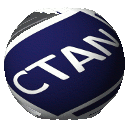# Directory `fonts/mathabx`

```Mathabx fonts series
--------------------

Last modification: May 16, 2005
---------------------------------

Summary
-------

The mathabx series are a large mathematical symbols set designed and
defined in MetaFont language. Many of common and uncommon symbols can
be found in these series. Encoding, metrics, designs are not supposed
to be frozen since improvements, changes of mind can always
happen. These programs are intended to produce bitmap fonts and a lot
of care is given about rasterization.

Brief description of the fonts
------------------------------

mathaXX (XX ranges from 5 to 12): quite common mathematical symbols;

mathbXX (XX ranges from 5 to 12): quite uncommon mathematical symbols;

mathxXX (XX ranges from 5 to 12): large and extensible mathematical
symbols which fit the two previous series;

mathcXX (XX ranges from 10 to 10): unsupported (yet) since it is an
incomplete calligraphic series;

mathuXX (XX ranges from 10 to 10): unsupported (forever) since it is a
too strange symbols series;

mathuxXX (XX ranges from 10 to 10): unsupported (forever) since it is
a too strange large or extensible symbols series.

Suggested TDS location for all ``.mf'' files:
---------------------------------------------
\$TEXMF/fonts/source/public/mathabx

Brief description of the (La)TeX files
--------------------------------------

mathabx.tex: ``plain TeX'' input file which defines the 3 families
matha (5,7,10), mathb (5,7,10) and mathx (10,10,10) then read
mathabx.dcl;

mathabx.sty: LaTeX input file which defines the 3 families matha,
mathb and mathx according to LaTeX fonts selection scheme, then read
mathabx.dcl;

mathabx.dcl: declaration of every new or conpound symbol.

Suggested TDS location: \$TEXMF/tex/generic/misc/
-----------------------

Support for installation: Almost none.
-------------------------

Documentation: mathtest.tex, testmac.tex.
--------------

mathtest.tex is a plain tex file that uses testmac as a macro file and which need that all the fonts should be properly installed. See postscript (or PDF) outputs provided in this distribution.

Brief commentary: Happy TeXing.
-----------------

Anthony Phan.
```

Download the contents of this package in one zip archive (939.5k).

## mathabx – Three series of mathematical symbols

Mathabx is a set of 3 mathematical symbols font series: matha, mathb and mathx. They are defined by code and should be of reasonable quality (bitmap output). Things change from time to time, so there is no claim of stability (encoding, metrics, design).

The package includes Plain and support macros.

A version of the fonts, in Adobe Type 1 format, is also available.

 Package mathabx Home page http://www-math.univ-poitiers.fr/~phan/ Licenses The LaTeX Project Public License Maintainer Anthony Phan Contained in TeX Live as mathabxMiKTeX as mathabx Topics Font symbol mathsMF FontFont